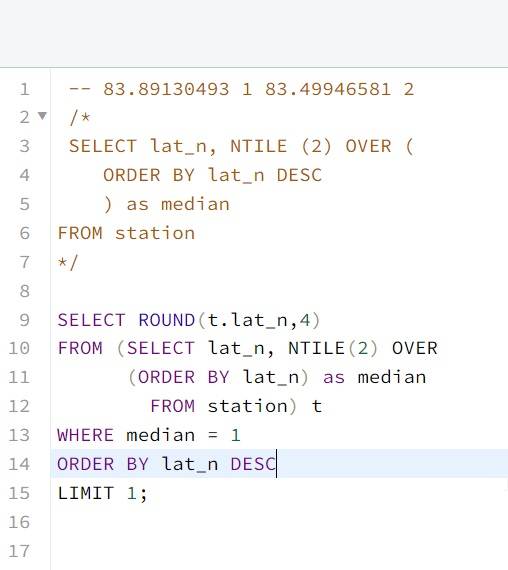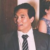# Hackerrank – SQL – Aggregation – Weather Observation Station 20 – Solution

Publish Date - November 8th, 2022

|

This was a really fun question, with a lot of moving parts. It tests your knowledge of basic statistics, while also looking to see if you can use multiple functions.

## The Question

median is defined as a number separating the higher half of a data set from the lower half. Query the median of the Northern Latitudes (LAT_N) from STATION and round your answer to  decimal places.

Input Format

The STATION table is described as follows:

where LAT_N is the northern latitude and LONG_W is the western longitude.

## The Solution

`````` -- 83.89130493 1 83.49946581 2
/*
SELECT lat_n, NTILE (2) OVER (
ORDER BY lat_n DESC
) as median
FROM station
*/

SELECT ROUND(t.lat_n,4)
FROM (SELECT lat_n, NTILE(2) OVER
(ORDER BY lat_n) as median
FROM station) t
WHERE median = 1
ORDER BY lat_n DESC
LIMIT 1;
``````

This solution is unlike a lot of the other ones I’ve posted, since it requires a good understanding on what a “median” is in statistics + a good understanding of intermediate MySQL commands.

So the question is:

median is defined as a number separating the higher half of a data set from the lower half. Query the median of the Northern Latitudes (LAT_N) from STATION and round your answer to  decimal places.

Basically, I read this as the following – “I need to split the results of Lat_N into two groups so that I can find the highest result in the first group. This with the understanding that the groups are ordered by DESC, and group 1 is the smaller sample size than group 2.”.

With that understanding I decided to use NTILE(2) MySQL command to split the group into two cohorts, and then ORDER BY Lat_N. Remember that NTILE() functions similar to a PARTITION() and you can give NTILE() values in the OVER() command to break up your cohorts differently.

Splitting the Lat_n into two groups to find the median.

``````SELECT lat_n, NTILE (2) OVER (
ORDER BY lat_n DESC
) as median
FROM station  ``````

This query will return all of the lat_n with a virtual column being 1 or 2, representing the cohorts outlined by NTILE sorted by lat_n descending (Cohort 2 will have bigger numbers than cohort 1). You’ll see a return value similar to below.

As you’ll see you’ll have 500 rows, which means you’ll want row 250 record (since 500 / 2 is 250). The median therefore is 83.8913.

In my solution, you can see that I added a comment to denote what the answer is – so I can work towards it.

`` -- 83.89130493 1 83.49946581 2``

Now with this query, I have what I need and I just need to clean it up.

I realized I had two problems:

1. I need a way to query only one cohort so that I can sort by descending and then isolate the value 83.8913 (remember I need to ROUND to the nearest 4 digits).
2. I need to someone drop the second column I’ve generated with NTILE(), as the unit tests for this problem won’t accept 2 values (one being lat_n and cohort).

Both of these issues can be solved with a subquery.

A subquery will allow me to run a WHERE clause and ask for only one cohort to be generated since now I’m able to constrain the query’s query.

It will also allow me to not need a second column (while having both columns existing).

``````SELECT ROUND(t.lat_n,4) -- ROUND to the 4 decimal point, with the subquery alias applied to lat_n
FROM (SELECT lat_n, NTILE(2) OVER
(ORDER BY lat_n) as median
FROM station) t -- Subquery of my NTILE, ordered highest to lowest, with an alias for median
WHERE median = 1 -- Only call the medians that are 1, so that I get the first 250 rows
ORDER BY lat_n DESC -- prioritize the highest value of lat_n because it's the number we want
LIMIT 1; -- Return one value.``````

## Conclusion

This is it! I loved this question since it had so many moving parts. Comparing other answers online, I’ve also solved it much different than many of the other folks out there. While a lot of them used FLOOR and CEILING to solve this issue, I believe my query is faster and more light weight than theirs.

### 2 thoughts on “Hackerrank – SQL – Aggregation – Weather Observation Station 20 – Solution”

1.SELECT ROUND(sub.LAT_N,4)
FROM (SELECT LAT_N
FROM STATION
ORDER BY LAT_N
LIMIT 250)sub
ORDER BY LAT_N DESC
LIMIT 1

Note: the limit is determine by first of all determine the total no of data in the LAT_ N using
SELECT COUNT(LAT_N)
FROM STATION
The result display = 499
The median formula is (n +1)/2 item of the data
Therefore, the Limit is determine by (499+1)/2 = 250

1.Hey Clement,

Interesting approach! Thanks for sharing!